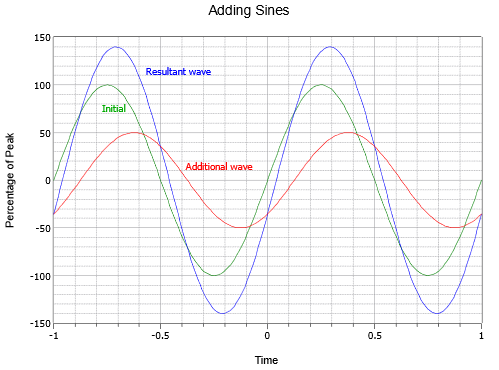# 9.6: Phasor Diagrams

$$\newcommand{\vecs}{\overset { \rightharpoonup} {\mathbf{#1}} }$$ $$\newcommand{\vecd}{\overset{-\!-\!\rightharpoonup}{\vphantom{a}\smash {#1}}}$$$$\newcommand{\id}{\mathrm{id}}$$ $$\newcommand{\Span}{\mathrm{span}}$$ $$\newcommand{\kernel}{\mathrm{null}\,}$$ $$\newcommand{\range}{\mathrm{range}\,}$$ $$\newcommand{\RealPart}{\mathrm{Re}}$$ $$\newcommand{\ImaginaryPart}{\mathrm{Im}}$$ $$\newcommand{\Argument}{\mathrm{Arg}}$$ $$\newcommand{\norm}{\| #1 \|}$$ $$\newcommand{\inner}{\langle #1, #2 \rangle}$$ $$\newcommand{\Span}{\mathrm{span}}$$ $$\newcommand{\id}{\mathrm{id}}$$ $$\newcommand{\Span}{\mathrm{span}}$$ $$\newcommand{\kernel}{\mathrm{null}\,}$$ $$\newcommand{\range}{\mathrm{range}\,}$$ $$\newcommand{\RealPart}{\mathrm{Re}}$$ $$\newcommand{\ImaginaryPart}{\mathrm{Im}}$$ $$\newcommand{\Argument}{\mathrm{Arg}}$$ $$\newcommand{\norm}{\| #1 \|}$$ $$\newcommand{\inner}{\langle #1, #2 \rangle}$$ $$\newcommand{\Span}{\mathrm{span}}$$

A time domain representation of sine waves as drawn earlier tells us everything we need to know about the waves, however it is not the most compact method of displaying them, nor are they easy to sketch by hand. Consider the the plot of Figure $$\PageIndex{1}$$. Here, two sine waves (green and red) combine to create a third sine wave (blue). While the general idea of the two waves adding is apparent in the graph, it takes a moment of inspection to determine the wave magnitudes and the precise phase relationships between them. In contrast, phasor diagrams can be used to show the relationships of multiple sines waves in a simple and easy to read format. They can also be used to show how various voltages or currents combine.Figure $$\PageIndex{1}$$: A time domain plot.

The phasor diagram is based on the complex plane discussed previously where the horizontal is the real axis and the vertical is the imaginary ($$j$$) axis. The magnitude and phase of each wave can then be drawn as a vector, and the relationships between the waves is shown directly. For manual plotting, it is convenient to convert from polar form to rectangular form. In the example phasor diagram of Figure $$\PageIndex{2}$$, two vectors are shown: $$8 + j6$$ and $$5 − j3$$ (equivalent to $$10\angle 36.9^{\circ}$$ and $$5.83\angle −31^{\circ}$$). Phasor diagrams can be used to plot voltages, currents and impedances. We shall make considerable use of them in upcoming chapters.Figure $$\PageIndex{2}$$: A phasor diagram.

9.6: Phasor Diagrams is shared under a CC BY-NC-SA license and was authored, remixed, and/or curated by James M. Fiore.